# Linear Regression

## Linear Regression is the beginner’s algorithm for a kick-start in Machine Learning. Let's take a deep dive into the Math behind this algorithm.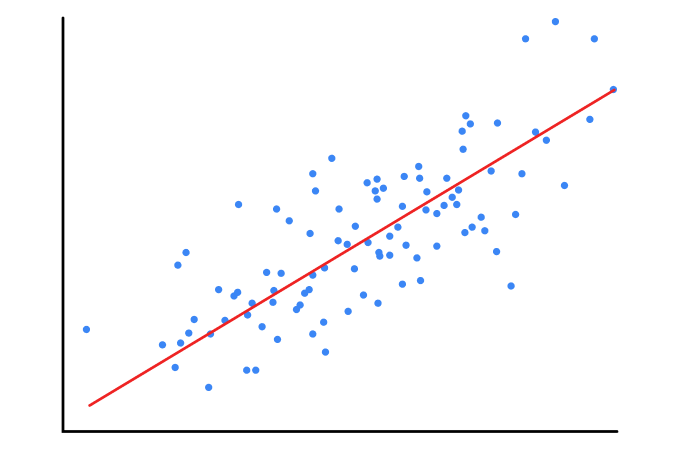Source: Author

Whenever you come across linear regression, the first thing that should come to your mind is a scatter plot image somewhat like this.

To set up the possible relationship among different variables, various modes of statistical approaches are implemented, known as regression analysis. To understand how the variation in an independent variable can impact the dependent variable, regression analysis is specially molded out.

Basically, regression analysis sets up an equation to explain the significant relationship between one or more predictors and response variables and also to estimate current observations. The regression outcomes lead to the identification of the direction, size, and analytical significance of the relationship between predictor and response, where the dependent variable could be numerical or discrete in nature.

# Where do we use this algorithm?

1. Hours spent studying Vs Marks scored by students
2. Amount of rainfall Vs Agricultural yield
3. Electricity usage Vs Electricity bill
4. Suicide rates Vs Number of stressful people
5. Years of experience Vs Salary
6. Demand Vs Product price
7. Age Vs Beauty
8. Age Vs Health issues
9. Number of Degrees Vs Salary
10. Number of Degrees Vs Education expenditure

So you may note that this is a widely used algorithm. Also, many other algorithms are derived from this algorithm.

# Types of regression techniques

In addition to it, the types of regression analysis can be selected on the attributes, target variables, or the shape and nature of the regression curve that reveal the relationship between dependent and independent variables. In this blog, we will discuss linear regression with MATH in Detail.

# Introduction

Linear Regression is a supervised machine learning algorithm where the predicted output is continuous and has a constant slope. It’s used to predict values within a continuous range (e.g., sales price) rather than trying to classify them as categories (e.g., cat, dog). There are two main types:

Simple regression

Simple linear regression uses a traditional slope-intercept form, where m and b are the variables. The algorithm will try to “learn” to produce the most correct predictions where x represents our input data and y represents our prediction.

`y = mx + b ; m: slope, b: intercept`

Multivariable regression

A more complex multi-variable linear equation might look like this, where we represent the coefficients or weights our model will try to learn.

`f(x,y,z) = w1x + w2y + w3z;x, y, z are three input parameters & w represents weight`

The variables x, y, z represent the attributes or distinct pieces of information we have about each observation. For sales predictions, these attributes might include a company’s advertising spend on radio, TV, and newspapers.

`Sales = w1Radio + w2TV + w3News`

# Simple Regression

Let’s say we are given a dataset with the following columns (features): how much a company spends on Radio advertising each year, vs. its annual sales of units sold. We are trying to develop an equation that will let us predict units sold based on how much a company spends on radio advertising. The rows (observations) represent companies.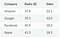Data Frame

# Making Prediction

The prediction function outputs an estimate of sales given a company’s radio advertising spend and our current values for Weight and Bias.

`Sales = Weight⋅Radio + Bias`

Weight: the coefficient for the Radio independent variable. In machine learning, we call coefficients weights.

Radio: the independent variable. In machine learning, we call these variables features.

Bias: the intercept where our line intercepts the y-axis. In machine learning, we can call intercepts bias. Bias offsets all predictions that we make.

Our aim is to achieve the best values for weight and bias and to achieve that we will use different techniques. By the end of our training, our equation will approximate the line of best fit. For updating these weight and biases, we will introduce a cost function (or loss function) and try to reduce it’s value (loss value).

# The Cost Function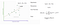COST FUNCTION (Source: here)

The main set-up for updating weight and biases is to define a cost function (also known as a loss function) that measures how well the model predicts outputs on the test set. The goal is to then find a set of weights and biases that reduces the value of the cost function. One common method that is often used is the mean squared error (MSE) to measure the difference between the actual value of y and the estimated value of y (predicted value). The equation of the above regression line is:

`hθ(x) = θ0 + θ1x (resembles y = mx + c)`

It has only two parameters: weight (θ1)and bias (θ0). This equation is used to make a cost-function equation, as seen in the above image. Further, the cost function is calculated multiple times till its value is decreased (up to some extent) and we get acceptable results. To compare the real value vs. predicted value, we either use MSE or variance.

Given our simple linear equation y=mx + b, we can calculate MSE as:

`MSE = (1/N) ∑(i=1 to n)(yi − (mxi + b))^2N is the total number of observations (data points)(1/N) ∑(i=1 to n) is the meanyi is the actual value of an observation and mxi+b is our prediction`

Are Variance and MSE the same?

Variance is the measure of how far the data points are spread out, whereas MSE is the measure of how the predicted values are different from the actual values. Though both are the measures of the second moment, there is a significant difference. In general, the sample variance measures the spread of the data around the mean (in squared units), while the MSE measures the vertical spread of the data around the regression line (in squared vertical units). Hope you don’t get confused by these terms. Further, to reduce the MSE, we perform gradient descent.

# Gradient Descent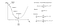GRADIENT DESCENT (Source: here)

To reduce MSE, we use Gradient Descent and calculate the gradient of our cost function. Gradient Descent runs iteratively to find the optimal values of the parameters corresponding to the decreasing value of the given cost function using calculus. Mathematically, the technique of the ‘derivative’ is extremely important to reduce the cost function because it helps get the minimum point. The derivative is a concept from calculus and refers to the slope of the function at a given point. We need to know the slope so that we know the direction (sign) to move the coefficient values to get a lower cost on the next iteration.

Math

There are two parameters (coefficients) in our cost function we can control: weight m and bias b. Since we need to consider the impact each one has on the final prediction, we use partial derivatives. To find the partial derivatives, we use the Chain Rule. We need the chain rule because (y−(mx+b))² is the two nested functions: the inner function (y−(mx+b)) and the outer function x².

Returning to our cost function: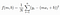COST FUNCTION

First, we will look at Chain Rule: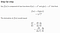CHAIN RULE

Based on this chain rule, we can calculate the gradient of this cost function as: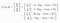Applying Gradient Descent to a Cost function

At this point, you must be thinking about how to code these functions. Relax!!

We don’t code these functions, instead, we directly import a linear regression module from the sci-kit learn library that automatically calculates the weights and biases. The sample code is shown below.

`#First splitting data for training data(85%) and testing data(15%)#test_size can be changed #random_state Controls the shuffling applied to the data before applying the split 1==True#Here x_data = df['Radio (\$)'] & y_data = df['Sales']from sklearn.model_selection import train_test_splitx_train, x_test, y_train, y_test = train_test_split(x_data, y_data, test_size=0.15, random_state=1)#Creating linear regression modelfrom sklearn.linear_model import LinearRegression#Create the linear regression objectlm = LinearRegression()lm.fit(x_train, y_train)test_y_hat = lm.predict(x_test)print("Mean absolute error: %.2f" % np.mean(np.absolute(test_y_hat - y_test)))print("Residual sum of squares (MSE): %.2f" % np.mean((test_y_hat - y_test) ** 2))print("Accuracy of train dataset is : ",lm.score(x_train,y_train))print("Accuracy of test dataset is : ",lm.score(x_test,y_test))`

# Multivariable regression

Let’s say we are given data on TV, radio, and newspaper advertising spend for a list of companies, and our goal is to predict sales of units sold.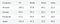DataFrame

# Normalization

As the number of features grows, calculating gradient takes longer to compute. We can speed this up by “normalizing” our input data to make sure all values are within the same range. This is especially important for datasets with high-standard deviations or differences in the ranges of the attributes. Our goal now will be to normalize our features so they are all in the range of -1 to 1.

# Making Prediction

Our predict function outputs an estimate of sales given our current weights (coefficients) and a company’s TV, radio, and newspaper spend. Our model will try to identify weight values that most reduce our cost function.

Sales = w1TV + w2Radio + w3Newspaper

# Cost Function

Now, we need a cost function to audit how our model is performing. The math is the same, except we swap the mx+b expression for w1x1+w2x2+w3x3. We also divide the expression by two to make derivative calculations simpler.

`MSE = (1/2N) (∑i=1n(yi−(W1x1+W2x2+W3x3))^2)`

# Gradient Descent

Again, using the Chain Rule, we can compute the gradient–a vector of partial derivatives describing the slope of the cost function for each weight.

`f′(W1)=−x1(y−(W1x1+W2x2+W3x3))f′(W2)=−x2(y−(W1x1+W2x2+W3x3))f′(W3)=−x3(y−(W1x1+W2x2+W3x3))`

# Simplifying the Matrix

The gradient descent code above has a lot of duplication. Can we improve it somehow? One way to refactor would be to loop through our features and weights–allowing our function to handle any number of features. But there is another, even better technique called vectorized gradient descent.

We use the same formula as above, but instead of working on a single feature at a time, we use matrix multiplication to operative on all features and weights simultaneously. We replace the xi terms with a single feature matrix X.

`gradient= −X(targets−predictions)`

This is how math works in multivariable regression. Understanding this much is more than enough because we never use it directly in our code. We use the algorithm directly using the sci-kit learn library.

I hope you liked this blog. Please make a feedback response.

Learning and exploring this beautiful world with amazing tech.

## More from Deep Patel

Learning and exploring this beautiful world with amazing tech.